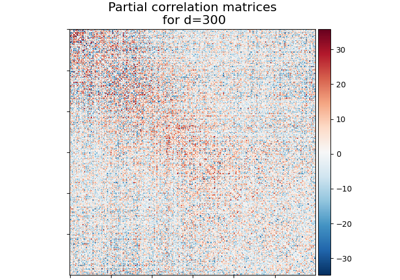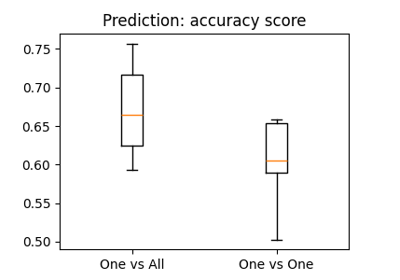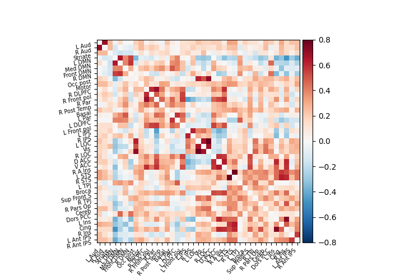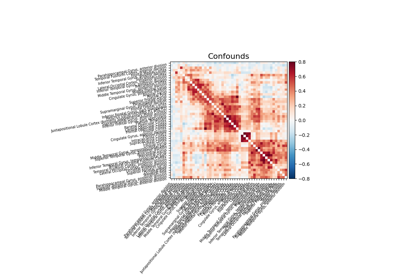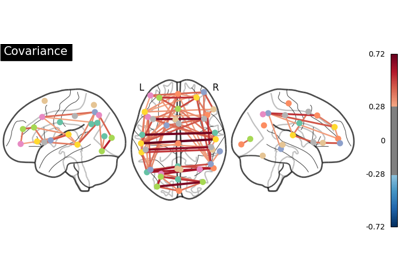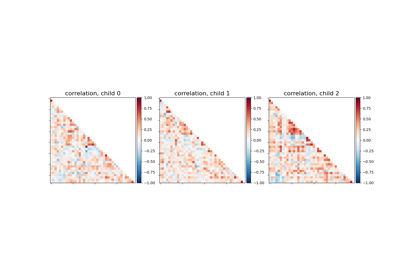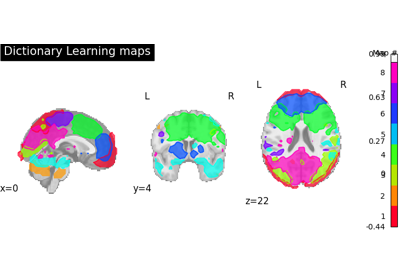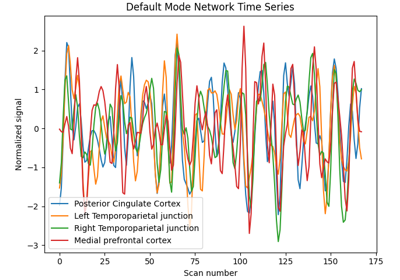Note

This page is a reference documentation. It only explains the function signature, and not how to use it. Please refer to the user guide for the big picture.

# 8.10.8. nilearn.plotting.plot_matrix¶

nilearn.plotting.plot_matrix(mat, title=None, labels=None, figure=None, axes=None, colorbar=True, cmap=<matplotlib.colors.LinearSegmentedColormap object>, tri='full', auto_fit=True, grid=False, reorder=False, **kwargs)[source]

Plot the given matrix.

Parameters
mat2-D numpy array

Matrix to be plotted.

title`str`, or None, optional

The title displayed on the figure. Default=None.

labelslist, ndarray of strings, empty list, False, or None, optional

The label of each row and column. Needs to be the same length as rows/columns of mat. If False, None, or an empty list, no labels are plotted.

figurefigure instance, figsize tuple, or None, optional

Sets the figure used. This argument can be either an existing figure, or a pair (width, height) that gives the size of a newly-created figure. Specifying both axes and figure is not allowed.

axesNone or Axes, optional

Axes instance to be plotted on. Creates a new one if None. Specifying both axes and figure is not allowed.

colorbar`bool`, optional

If `True`, display a colorbar on the right of the plots. Default=True.

cmap`matplotlib.colors.Colormap`, or `str`, optional

The colormap to use. Either a string which is a name of a matplotlib colormap, or a matplotlib colormap object. Default=`plt.cm.RdBu_r`.

tri{‘full’, ‘lower’, ‘diag’}, optional

Which triangular part of the matrix to plot: ‘lower’ is the lower part, ‘diag’ is the lower including diagonal, and ‘full’ is the full matrix. Default=’full’.

auto_fitboolean, optional

If auto_fit is True, the axes are dimensioned to give room for the labels. This assumes that the labels are resting against the bottom and left edges of the figure. Default=True.

gridcolor or False, optional

If not False, a grid is plotted to separate rows and columns using the given color. Default=False.

reorderboolean or {‘single’, ‘complete’, ‘average’}, optional

If not False, reorders the matrix into blocks of clusters. Accepted linkage options for the clustering are ‘single’, ‘complete’, and ‘average’. True defaults to average linkage. Default=False.

Note

This option is only available with SciPy >= 1.0.0.

New in version 0.4.1.

kwargsextra keyword arguments, optional

Extra keyword arguments are sent to pylab.imshow.

Returns
displayinstance of matplotlib

Axes image.

## 8.10.8.1. Examples using `nilearn.plotting.plot_matrix`¶